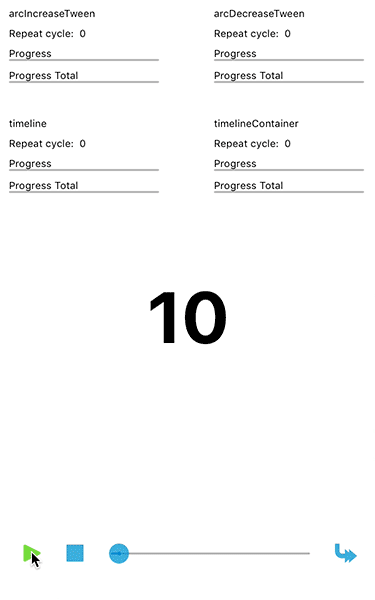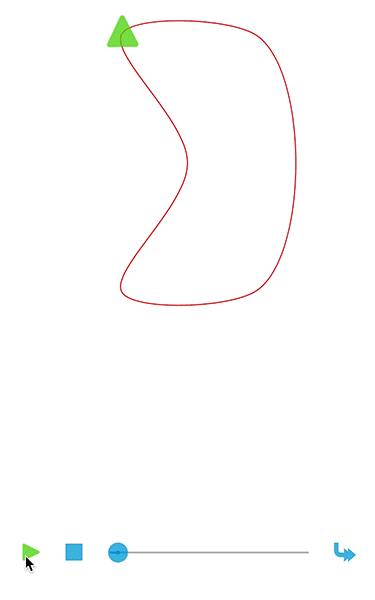Latest 1.0 https://github.com/RobinFalko/Ubergang Apache ios 8.0, requires ARC Foundation Robin FrielingsdorfUbergang is a tweening engine for iOS written in Swift.

## Features

• [x] Tween numeric values, UIColors and CGAffineTransforms
• [x] Tween along UIBezierPaths
• [x] Tween through points
• [x] Linear, Expo, Cubic, Quad, Circ, Quart, Quint, Sine, Back, Bounce and Elastic easings
• [x] Generic tween setup
• [x] Repeat and Yoyo tween options
• [x] Memory management for strong and weak tween object references
• [x] Tween Timelines
• [x] Bezier tween align to path
• [x] Logging and log levels

## Previews## Installation

### CocoaPods

``````    platform :ios, '8.0'
use_frameworks!
pod 'Ubergang'``````

## Setup

``````    UTweenSetup.instance.enableLogging(true)
UTweenSetup.instance.enableLogging(true, withLogger: loggerProxy)``````

Ubergang provides some logs for basic operations like start, stop, pause, …
There is a dependency to XCGLogger which is used by default, but you can pass any Logger you prefer by creating a custom logger proxy implementing `UTweenLoggable`.

## Tween Configuration

``````    .options(.repeat(n))
.options(.yoyo)
.options(.repeat(n), .yoyo)``````

Using `options` you can let the Tween repeat n (Int) times, let it yoyo or combine both options.

• Repeat will restart the Tween n times where `repeatCycleChange` will be called with every cycle.
• Yoyo will reverse the Tween after it reaches the end value.
``````    .reference(.strong)` //(default)
.reference(.weak)``````

`reference` determines how to handle the reference count for the tween. Ubergang will increase the retain count if the option is set to `.strong` or won’t increase it if it’s set to `.weak`. These two rules are valid for most cases:

• The Tween is not stored in a field variable -> `.strong`
• The Tween is stored in a field variable -> `.weak`

## Usage

### Start a simple numeric Tween (Double)

``    0.tween(to:10).update({ (value:Int) in print("(value)") }).start()``

This Tween goes from 0 to 10 over 0.5 by default seconds using a linear easing by default. The current value will be printed with every update.

### ‘to’ and ‘from’ using closures

``````    NumericTween(id: "doubleTween")
.from({ [unowned self] in return self.position2.x }, to: { [unowned self] in return self.position1.x })
.update({ value, progress in print("update: (value), progress: (progress) ") })
.duration(5)
.start()``````

Passing closures to ‘to’ and ‘from’ will always compute all results using the current values returned by the closures.

### Start a weak numeric Tween (Int)

``````    var tween: NumericTween<Int>?

func run() {
tween = 0.tween(to: 10)
.id("intTween")
.duration(5)
.update({ value in print("update: (value)") })
.ease(Elastic.easeOut)
.reference(.weak)
.start()
}``````

This Tween with id ‘intTween’ goes from 0 to 10 over 5 seconds using an elastic easing. The current value will be printed with every update.
.reference(.weak) will store this tween weakly, Ubergang won’t increment the reference count. It’s up to you to keep the Tween alive.

### Start a numeric Tween repeating 5 times with yoyo

``````    var tween: NumericTween<Int>?

func run() {

tween = 0.tween(to: 10)
.id("intTween")
.duration(5)
.update({ value in print("update: (value)") })
.ease(Elastic.easeOut)
.reference(.weak)
.options(.repeat(5), .yoyo)
.start()
}``````

### Start a weak numeric Tween (CGAffineTransform)

``````    @IBOutlet var testView: UIView!
var tween: TransformTween?

func run() {
//declare the target values
var to = testView.transform
to.ty = 200.0

tween = testView.transform.tween(to: transform)
.id("testView")
.duration(2.5)
.reference(.weak)
.update({ [unowned self] value in self.testView.transform = value })
.start()
}``````

This Tween with id ‘testView’ tweens a transform over 2.5 seconds. The resulting tranform will be assigned to the testView with every update ‘welf.testView.transform = value’.

### Start a Timeline containing three Tweens

``````    var timeline: UTimeline = UTimeline(id: "timeline")

func run() {
timeline.options(.yoyo).reference(.weak)

timeline.append(
0.tween(to: 10).id("intTween").duration(5).update({ value, _ in print("0-10 value: (value)") })
)

timeline.append(
0.0.tween(to: 10.0).id("floatTween1").duration(5).update({ value, _ in print("0.0-10.0 value: (value)") })
)

timeline.insert(
10.0.tween(to: 0.0).id("floatTween2").duration(5).update({ value, _ in print("10.0-0.0 value: (value)") }), at: 2.5
)

timeline.start()
}``````

This Timeline controls one Tween starting at time 0.0 seconds, one Tween starting at time 5.0 seconds and the last one starting at 2.5 seconds. All Tweens are controlled by the timeline with the given options – In this case the tween option `.yoyo`

### Tween along a UIBezierPath

``````    var tween: BezierPathTween!

func run() {
tween = BezierPathTween().along(path)
.id("bezierTween")
.duration(5)
.ease(Linear.ease)
.reference(.weak)
.update({ [unowned self] (value: CGPoint, progress: Double) in
//update
})
.start()
}``````

### Tween through points

``````    var tween: BezierPathTween!

func run() {
let points = [CGPoint]()
points.append(...)

tween = BezierPathTween().along(points)
.id("bezierTween")
.duration(5)
.ease(Linear.ease)
.reference(.weak)
.update({ [unowned self] (value: CGPoint, progress: Double) in
//update
})
.start()
}    ``````

### Tween through points and use orientation to align the object on update

``````    var tween: BezierPathTween!

func run() {
let points = [CGPoint]()
points.append(...)

tween = BezierPathTween().along(points)
.id("bezierTween")
.duration(5)
.ease(Linear.ease)
.reference(.weak)
.update({ [unowned self] (value:CGPoint, progress: Double, orientation: CGPoint) in
self.targetView.center = value

let angle = atan2(orientation.y, orientation.x)
let transform = CGAffineTransformRotate(CGAffineTransformIdentity, angle)
self.targetView.transform = transform
})
.start()
}      ``````

## Changelog Verion 1.0

• Swift 4 migration
• Change tween creation pattern (UTweenBuilder removed – Instead instantiate the tween object directly or use the appropriate extension like `0.tween(to: 10)`)
• Fix issue where timelines didn’t work if there was a delay between tweens

Feedback is always appreciated

### Latest podspec

```{
"name": "Ubergang",
"version": "1.0",
"summary": "A tweening engine written in Swift.",
"homepage": "https://github.com/RobinFalko/Ubergang",
"type": "Apache",
},
"authors": {
"Robin Frielingsdorf": "[email protected]"
},
"source": {
"git": "https://github.com/RobinFalko/Ubergang.git",
"tag": "1.0"
},
"source_files": "Ubergang/**/*.{h,m,swift}",
"platforms": {
"ios": "8.0"
},
"frameworks": "Foundation",
"requires_arc": true,
"pushed_with_swift_version": "3.0"
}```